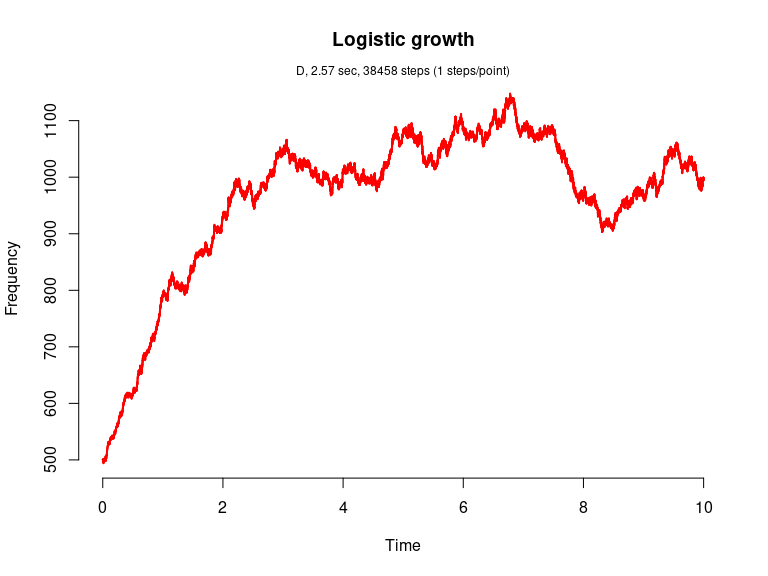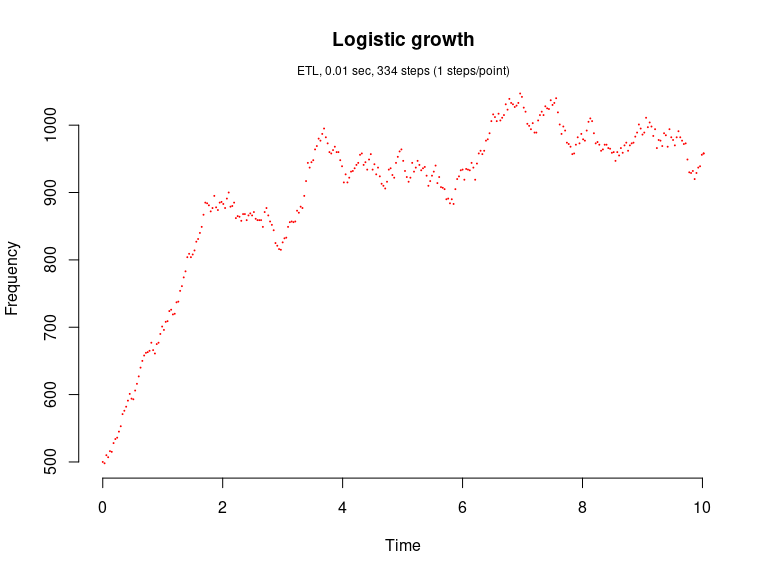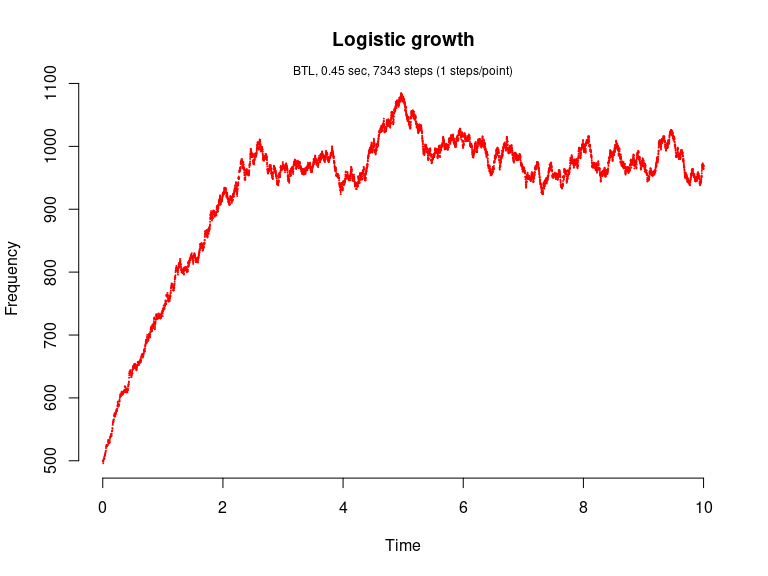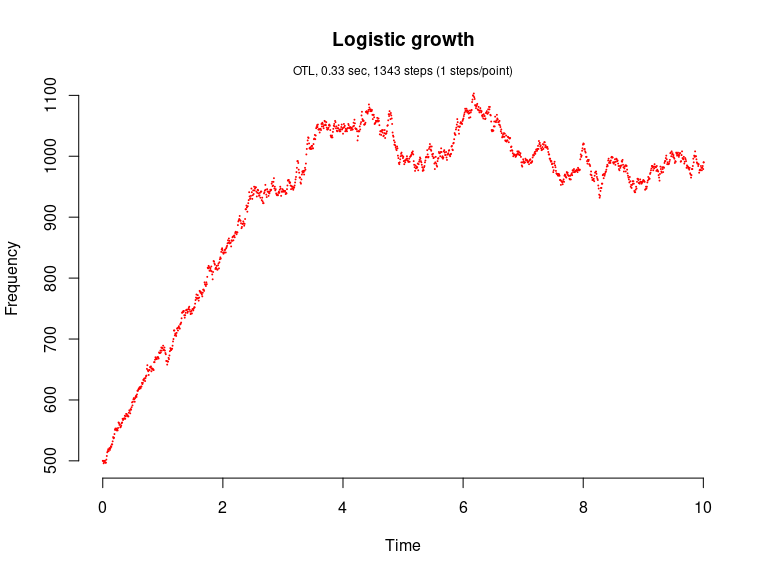# Pearl-Verhulst Logistic growth model (Kot, 2001)

The logistic growth model is given by dN/dt = rN(1-N/K) where N is the number (density) of indviduals at time t, K is the carrying capacity of the population, r is the intrinsic growth rate of the population. We assume r=b-d where b is the per capita p.c. birth rate and d is the p.c. death rate.

This model consists of two reaction channels,

 N ---b--->  N + N
N ---d'---> 0

where d'=d+(b-d)N/K. The propensity functions are a_1=bN and a_2=d'N.

library(GillespieSSA)

Define parameters

parms <- c(b = 2, d = 1, K = 1000)      # Parameters
tf <- 10                                # Final time
simName <- "Logistic growth" 

Define initial state vector

x0 <- c(N = 500)

Define state-change matrix

nu <- matrix(c(+1, -1),ncol = 2)

Define propensity functions

a  <- c("b*N", "(d+(b-d)*N/K)*N")

Run simulations with the Direct method

set.seed(1)
out <- ssa(
x0 = x0,
a = a,
nu = nu,
parms = parms,
tf = tf,
method = ssa.d(),
simName = simName,
verbose = FALSE,
consoleInterval = 1
)
ssa.plot(out, show.title = TRUE, show.legend = FALSE)Run simulations with the Explict tau-leap method

set.seed(1)
out <- ssa(
x0 = x0,
a = a,
nu = nu,
parms = parms,
tf = tf,
method = ssa.etl(tau = .03),
simName = simName,
verbose = FALSE,
consoleInterval = 1
)
ssa.plot(out, show.title = TRUE, show.legend = FALSE)Run simulations with the Binomial tau-leap method

set.seed(1)
out <- ssa(
x0 = x0,
a = a,
nu = nu,
parms = parms,
tf = tf,
method = ssa.btl(f = 5),
simName = simName,
verbose = FALSE,
consoleInterval = 1
)
ssa.plot(out, show.title = TRUE, show.legend = FALSE)Run simulations with the Optimized tau-leap method

set.seed(1)
out <- ssa(
x0 = x0,
a = a,
nu = nu,
parms = parms,
tf = tf,
method = ssa.otl(),
simName = simName,
verbose = FALSE,
consoleInterval = 1
)
ssa.plot(out, show.title = TRUE, show.legend = FALSE)